# High School Algebra - Linear Equations: Tutoring Solution Chapter Exam

Exam Instructions:

Choose your answers to the questions and click 'Next' to see the next set of questions. You can skip questions if you would like and come back to them later with the yellow "Go To First Skipped Question" button. When you have completed the practice exam, a green submit button will appear. Click it to see your results. Good luck!

### Page 1

#### Question 1 1. Which of these equations represents a line that is perpendicular to the line in the graph below?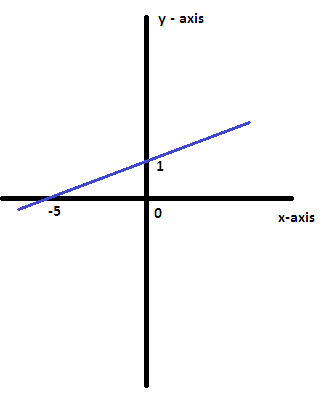#### Question 5 5. If the graph below has the equation Ax + By = -4, what must be true about A and B?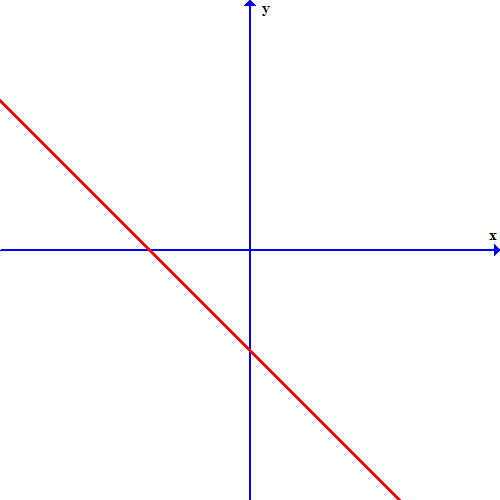### Page 2

#### Question 10 10. Which two lines are parallel?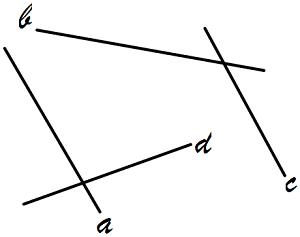### Page 3

#### Question 15 15. Which linear equation is shown below?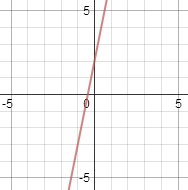### Page 4

#### Question 16 16. If the graph below was written in the slope-intercept form, which of the following would be true?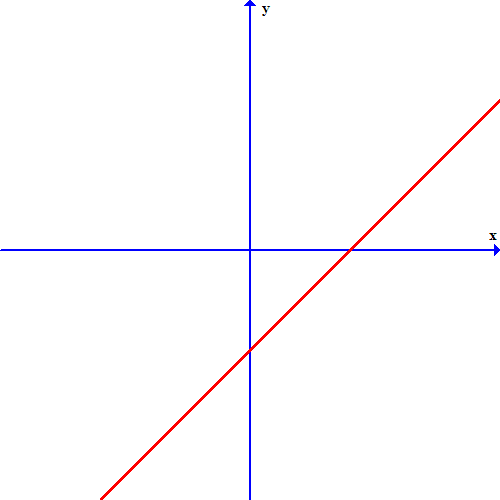#### Question 17 17. Which of the following is a solution to the system of equations shown in the graph below?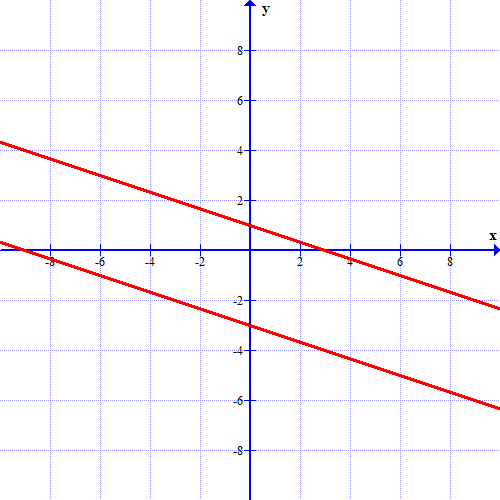### Page 6

#### High School Algebra - Linear Equations: Tutoring Solution Chapter Exam Instructions

Choose your answers to the questions and click 'Next' to see the next set of questions. You can skip questions if you would like and come back to them later with the yellow "Go To First Skipped Question" button. When you have completed the practice exam, a green submit button will appear. Click it to see your results. Good luck!

Support